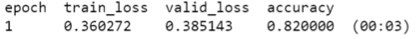# How are the validation and test set errors measured?Apologies for the very simplistic question, In this example here I understand that accuracy is measured from 0-1, but what is the scale for train/val errors, as I have seen them take a wide range of values.

No scale. They can be any value from 0 to infinite. These loss terms measure how similar your predicted and the actual output is. If both the outputs are similar you get a loss term close to 0 and if they are different you get higher loss values.

Fantastic cheers, so how are they calculated? RMSE ?

If it’s classification, we use Flattened Cross Entropy Loss which you can read more about (for the math) here